JS++ | Loops

• Last Updated : 03 Aug, 2018

It often happens in computer programming that you want a piece of code to execute more than once. Loops are programming statements that allow you to handle such cases. JS++ contains loops of various kinds, and we will look at several of them in this tutorial. We will begin, however, by examining two expressions that are used very commonly to facilitate the use of loops: the increment operator “++”, and the decrement operator “–“.

Note: The increment and decrement operators, and the loop statements that we will examine in this tutorial, work in a similar way to their counterparts in languages such as JavaScript, Java, C++, and C#.

“++” and “–“

To show these operators in action, let’s take an example. Make a new folder and name it “Loops”. Then make a new file and name it “Loops.jspp”. Write in the following code:

external \$;

int i = 0;
\$("#content").append("i is now ", i, "; ");
++i;
\$("#content").append("i is now ", i, "; ");
--i;
\$("#content").append("i is now ", i, "; ");

Save Loops.jspp to your Loops folder. Then create a second file named “Loops.html” and write in the following:

<!DOCTYPE html>
<title>Loops program</title>
<body>
<p id="content"></p>
<script src="http://code.jquery.com/jquery-1.12.4.min.js"></script>
<script src="Loops.jspp.js"></script>
</body>
</html>

Save Loops.html to your Loops folder. Compile Loops.jspp and then open Loops.html in a browser. Your document should show that “++” increases the value of i by 1, and “–” decreases i by 1.

As in various other programming languages, “++” and “–” can be used either before or after the variable. To illustrate the difference between these “prefix” and “postfix” uses, change the code in Loops.jspp to the following:

external \$;

int i = 0;
int j = 0;
j = ++i;
\$("#content").append("j is now ", j, ", and i is now ", i, "; ");
j = i++;
\$("#content").append("j is now ", j, ", and i is now ", i, "; ");

The variable i is incremented for the first time through the use of the prefix increment operator. This means that i is incremented before its value is used in the statement, and the value of j is therefore set to 1. When i is incremented for the second time, by contrast, it is through the use of the postfix increment operator. This means that i is incremented after its value is used in the statement, and the value of j therefore remains 1. The prefix / postfix distinction applies in exactly the same way to the two versions of the decrement operator.

“while” and “do… while”

Now let’s examine how we can use the increment and decrement operators in loops. We will look at three types of loops in this tutorial: while loops, do... while loops, and for loops. We begin with while loops.

The purpose of a while loop is to make some code – the loop body – execute repeatedly for as long as a particular condition is true. When the condition is no longer true, the loop body ceases to execute.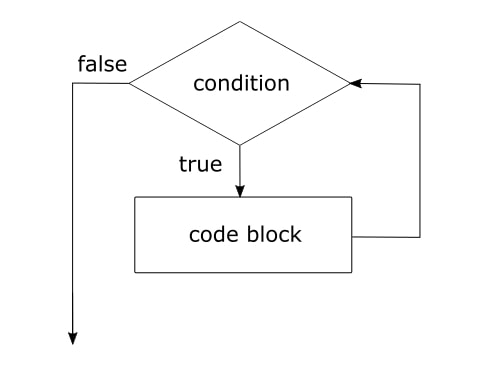Let’s take an example. Change the code in Loops.jspp to the following:

external \$;

int i = 0;
while (i < 3){
\$("#content").append("i is now ", i, "; ");
i++;
}

Here we see the syntax of a while loop: the while keyword, followed by a pair of parentheses holding a condition to be checked, followed by a block of code in curly braces which will execute repeatedly for as long as the condition is true.

In this example, the loop executes three times: once when i is 0, a second time when i is 1, and a third time when i is 2. During the third execution i is incremented to 3; this means that when the condition (i < 3) is next checked, it is found to be false and the loop terminates.

What happens if the condition in a while loop is false from the outset? If so, the loop body will not execute. Your program’s execution simply continues to the first statement following the loop. That might be exactly the behaviour you want; in other cases it might not be. If instead you want your loop to execute at least once before checking whether a condition is true, there is a different kind of loop for that purpose: the do... while loop.

Change the code in Loops.jspp to the following:

external \$;

int i = 0;
do {
\$("#content").append("i is now ", i, "; ");
i++;
}
while (i > 10);

In a do... while loop, the loop body is written after the do keyword, but before the condition to be checked. The loop executes at least once even if the condition is false on first time of checking, as it is in this example. When the condition is found to be false, the loop terminates.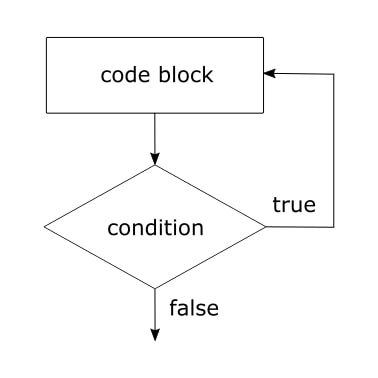“for”

for loops differ from while and do... while loops in one important respect: the syntax of a for loop is specifically designed to facilitate the use of counter variables. Let’s take a look at an example:

external \$;

for (int i = 0; i < 2; i++) {
\$("#content").append("i is now ", i, "; ");
}

The syntax of a for loop, as illustrated by this example, is somewhat more complex than that of a while or do... while loop so we will take a little more time to go over it. We can see that a for loop starts with the for keyword, which is then followed by some code in parentheses. The code in parentheses has three components, which are separated by semi-colons. The first part is the initialization component, where we declare our counter variable:

int i = 0;

The second part is the condition component, which provides a test to determine when the loop should terminate:

i < 3;

The third part is the update component, which would typically increment or decrement the counter variable:

i++

The code in parentheses is then followed by the loop body, in a pair of curly braces. The loop body is composed of the statement(s) to be executed.

A for loop proceeds as follows (see also the illustration below). First, the counter variable is initialized. Second, the condition is checked. If it is false then the loop terminates; if it is true then the loop continues to the next step. Third, the loop body executes. Fourth, the update component executes. Fifth, the loop returns to step two and repeats until the condition becomes false.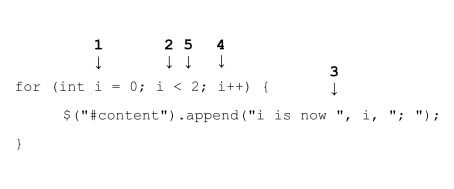In our example, the loop body executes twice: once when the counter variable i is 0, and again when i is 1. After the second execution, the update component increments i to 2. This means that when the condition i < 2 is checked, it is found to be false and the loop terminates.

Note: The initialization, condition, and update components of a for loop are all optional, strictly speaking, though one would normally expect to include them. For more information about non-standard uses, see:

JS++ for loops

“break” and “continue”

The break and continue keywords give you extra flexibility in using loops, and both can be used in all the types of loops that we’ve examined. It is important to understand the difference between break and continue, however: break is used to terminate a loop immediately, whereas continue is used to skip to the next iteration.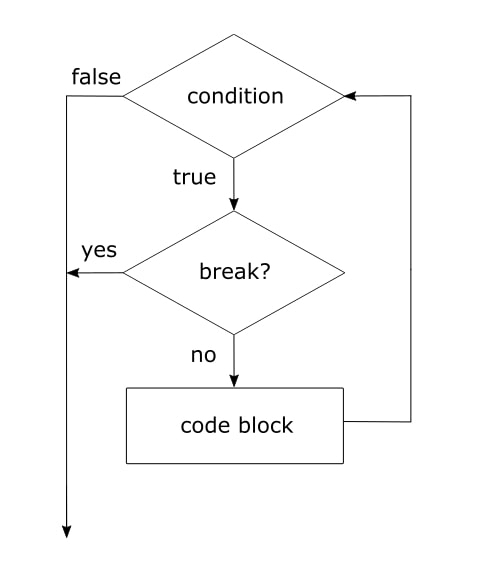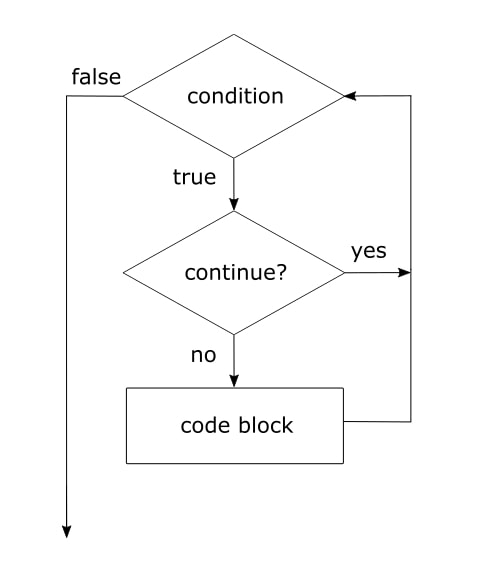To illustrate the difference, let’s take an example involving a while loop:

external \$;

int i = 0;
while (i < 3) {
i++;
if(i == 2) {
break;
}
\$("#content").append("i is now ", i, "; ");
}

If you write this code in to Loops.jspp, compile it, and then open Loops.html in a browser, it will display “i is now 1;”. It doesn’t display any more than that since the loop terminates midway through its second iteration, due to the use of break. If you remove break and replace it with continue, however, you will see a different result: Loops.html displays “i is now 1; i is now 3;”. This shows that continue doesn’t terminate the loop completely; it only terminates the current iteration, so that the loop skips ahead to the next iteration.

Nesting and labelled loops

Loops can be nested, which means putting one loop inside another. Here is an example of a nested for loop:

external \$;

for (int i = 0; i < 2; i++) {
\$("#content").append("i is now ", i, "; ");
for (int j = 0; j < 2; j++) {
\$("#content").append("j is now ", j, "; ");
}
}

In cases such as this, the inner loop runs through all of its iterations for each iteration of the outer loop. In this case, the outer loop executes twice and for each of those two executions, the inner loop executes twice. The inner loop therefore executes four times in total.

If you want to use break or continue in nested loops, it can be useful to label your loops. For example, consider this use of break in a nested loop:

external \$;

outer: for (int i = 0; i < 2; i++) {
\$("#content").append("i is now ", i, "; ");
inner: for (int j = 0; j < 2; j++) {
if(j == 0) {
break outer;
}
\$("#content").append("j is now ", j, "; ");
}
}

By labelling the outer loop outer, we make it possible to refer to that loop when using the break statement inside the inner loop. This use of break therefore terminates the outer loop, not just the inner one. If we had not used labels, break would have referred to the inner loop since by default break refers to the innermost loop in which it occurs. Parallel remarks apply to continue: by default it refers to the innermost loop in which it occurs, but it can also refer explicitly to an outer loop via a label.

My Personal Notes arrow_drop_up